# 【树】你真的会二叉树了嘛? --二叉树LeetCode专题Ⅲ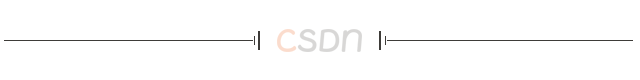Halo，这里是Ppeua。平时主要更新C语言，C++，数据结构算法......感兴趣就关注我吧！你定不会失望。

🌈个人主页：主页链接

🌈算法专栏：专栏链接

我会一直往里填充内容哒！

🌈LeetCode专栏：专栏链接

目前在刷初级算法的LeetBook 。若每日一题当中有力所能及的题目，也会当天做完发出

🌈代码仓库：Gitee链接

🌈点击关注=收获更多优质内容🌈

呜呜呜呜 今天被蓝桥杯爆杀了# 题目:100. 相同的树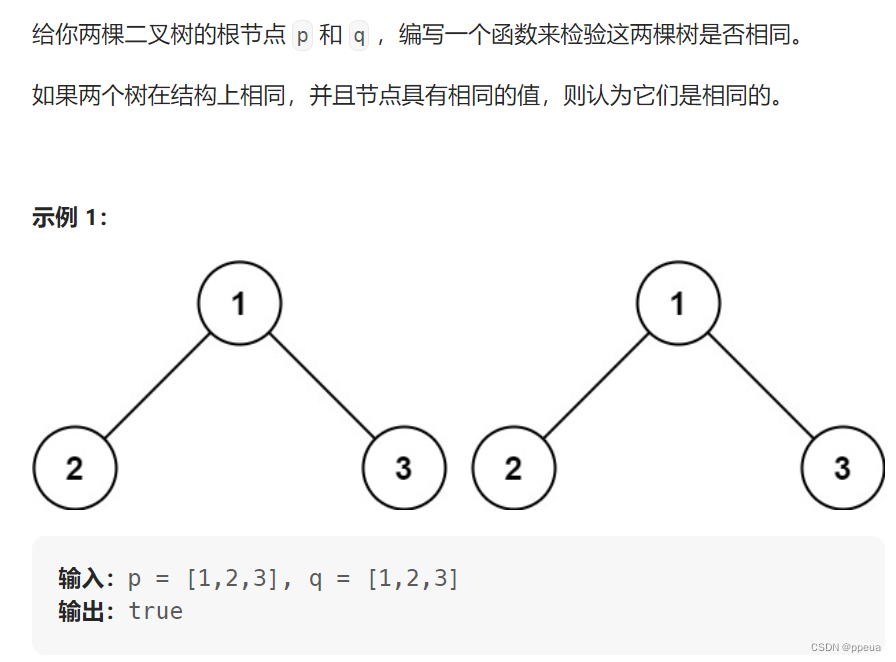# 题解: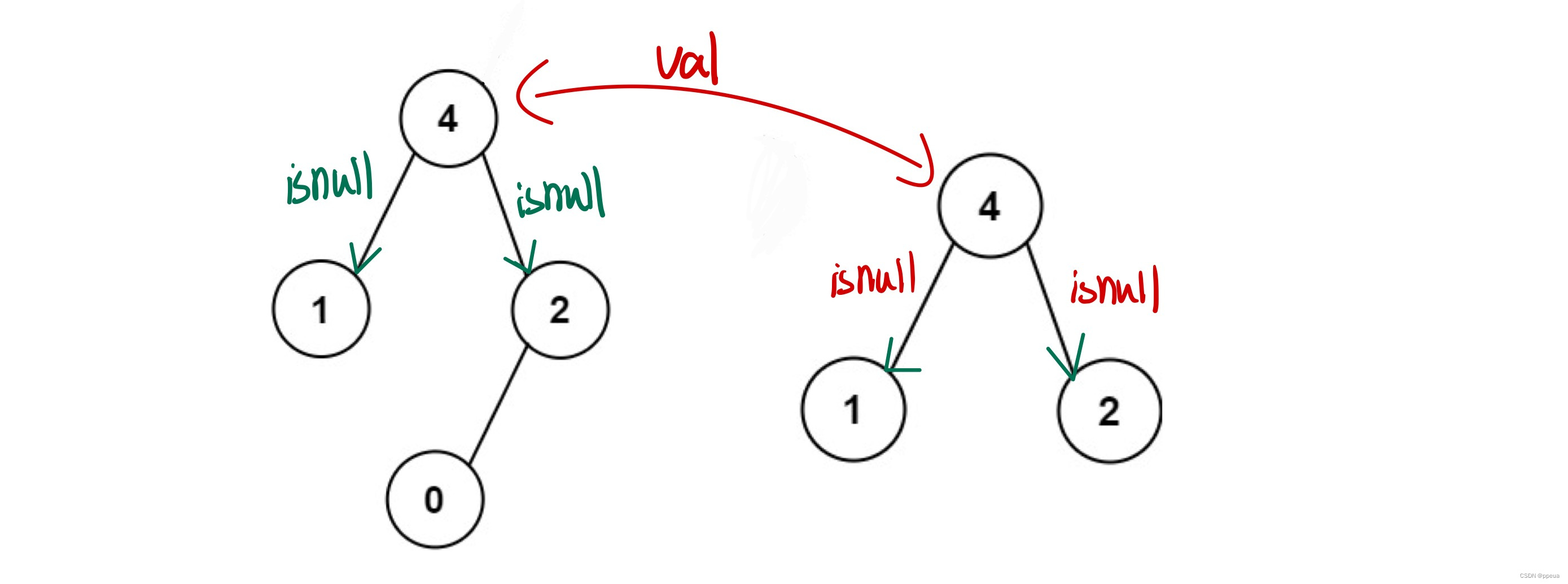## 代码实现:

``````
struct TreeNode {
int val;
struct TreeNode *left;
struct TreeNode *right;
};

#include<iostream>
using namespace std;
bool isSameTree(struct TreeNode* p, struct TreeNode* q){
if(p==NULL&&q==NULL)return true;
if(p==NULL||q==NULL)return false;
if(p->val!=q->val)return false;

return (isSameTree(p->left,q->left)&&isSameTree(p->right,q->right));

}   ``````

# 题目:572. 另一棵树的子树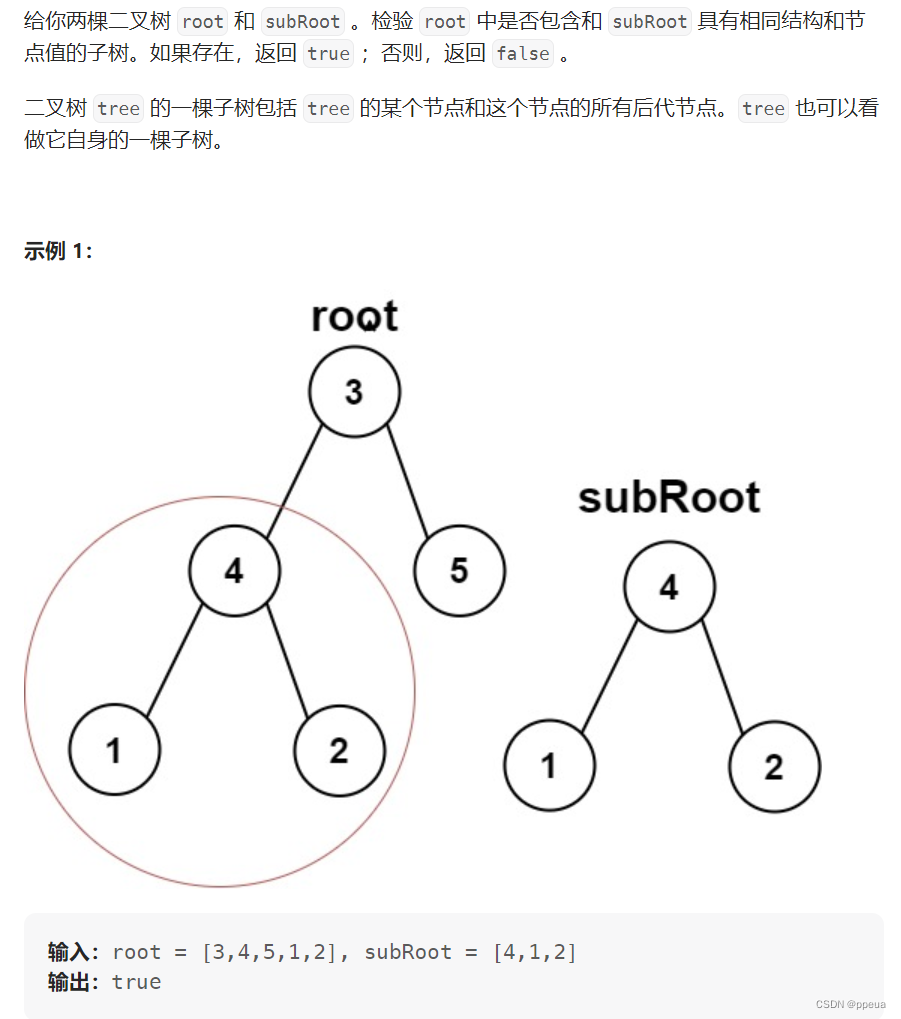# 题解:

## 代码实现:

``````#include<iostream>
using namespace std;
struct TreeNode {
int val;
struct TreeNode *left;
struct TreeNode *right;
};
bool isSameTree(struct TreeNode* p, struct TreeNode* q)
{
if(!p&&!q)return true;
if(!p||!q)return false;
if(p->val!=q->val)return false;
return isSameTree(p->left, q->left)&&isSameTree(p->right, q->right);
}

bool isSubtree(struct TreeNode* root, struct TreeNode* subRoot){
if(root==NULL)return false;
if(isSameTree(root, subRoot))return true;
return isSubtree(root->left,subRoot)||isSubtree(root->right, subRoot);
}``````

# 题目:236. 二叉树的最近公共祖先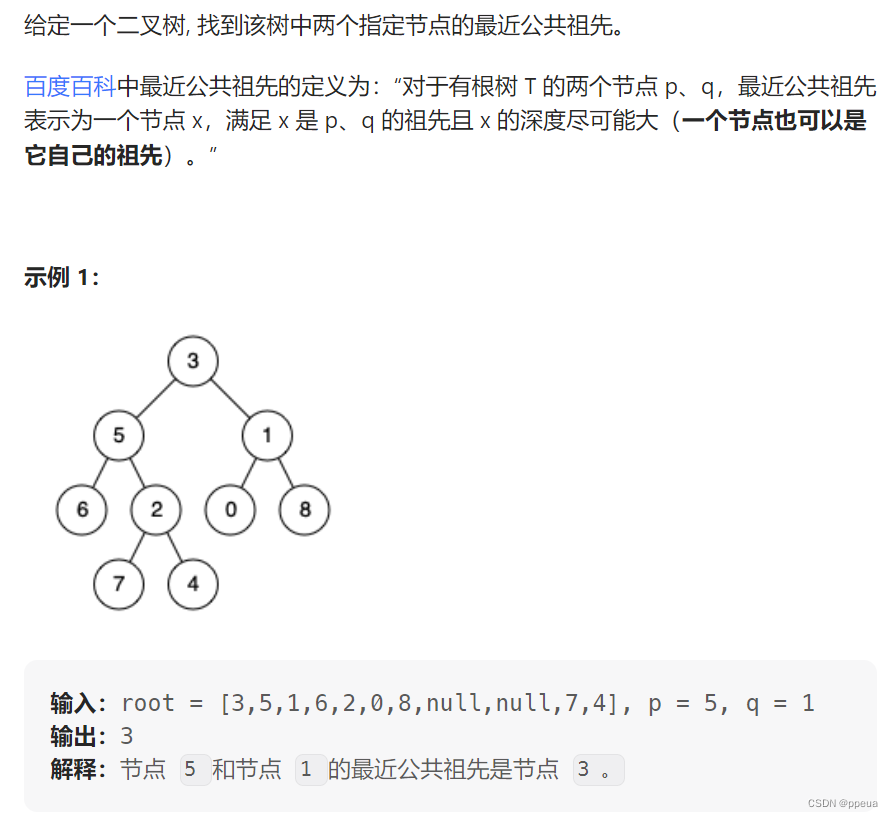# 题解:若只有一边找到了,则说明这其中一个节点可能做为另一个节点的根,则直接返回根节点(left,right)即可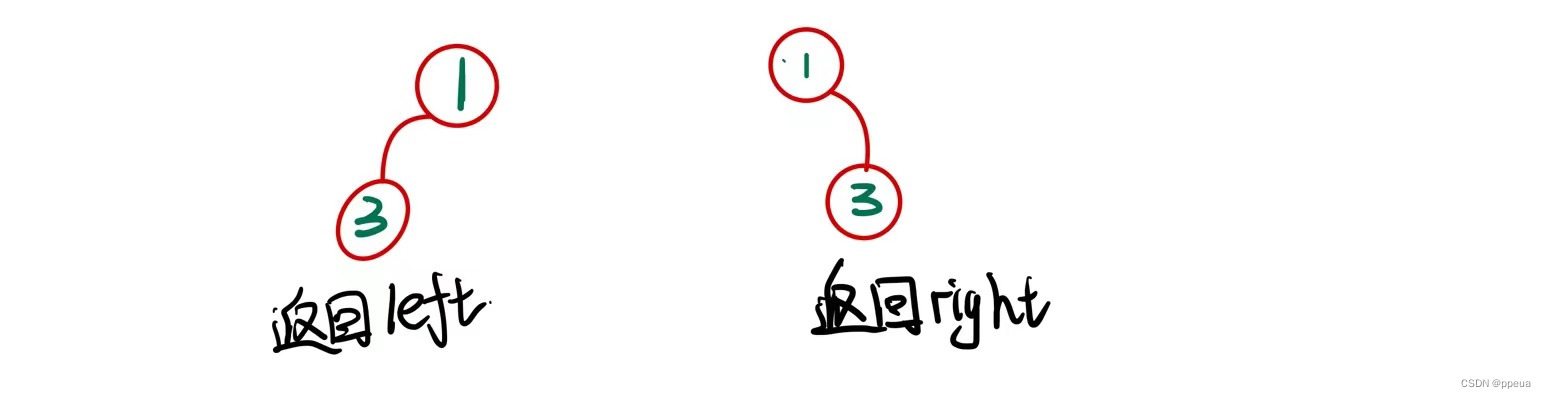​​​​​​​        ​​​​​​​     依次遍历访问每棵树的右节点称为right

## 代码实现:

``````#include<iostream>
using namespace std;
struct TreeNode {
int val;
TreeNode *left;
TreeNode *right;
};

class Solution {
public:
TreeNode* lowestCommonAncestor(TreeNode* root, TreeNode* p, TreeNode* q) {
if(root==NULL||root->val==p->val||root->val==q->val)return root;
TreeNode* left=lowestCommonAncestor(root->left,  p, q);
TreeNode*right=lowestCommonAncestor(root->right,p,q);
if(left!=NULL&&right!=NULL)return root;
if(right==NULL)return left;
if(left==NULL)return right;
return NULL;
}
};``````

# 完结撒花：

🌈本篇博客的内容【你真的会二叉树了嘛? --二叉树LeetCode专题Ⅲ】已经结束。

🌈若对你有些许帮助，可以点赞、关注、评论支持下博主，你的支持将是我前进路上最大的动力。

🌈若以上内容有任何问题，欢迎在评论区指出。若对以上内容有任何不解，都可私信评论询问。

🌈诸君，山顶见！